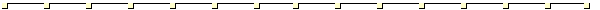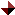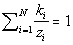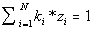Calculation TemperatureCondensation temperature can be calculate for the following equations :where

zi is the molar fraction of each component of the mixture.

ki is the equilibrium constant of each component.

This equation will be true when the temperature is the condensation temperature or closed.Boil temperature can be calculate for the following equations :where

zi is the molar fraction of each component of the mixture.

ki is the equilibrium constant of each component.

This equation will be true when the temperature is the boil temperature or closed.